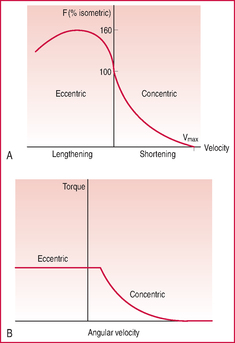# force-velocity relationshipForce-velocity relationship. (A) F-V curve including region of eccentric activity. (B) Torque-angular velocity curve for human knee extensors in both concentric and eccentric actions, obtained by isokinetic dynamometry.

## force-velocity relationship

the relationship between force and velocity in a contracting muscle or isolated muscle fibre. In concentric contraction force is zero at maximum velocity and maximal at very low or at zero velocity (the latter being an isometric contraction). Between these extremes the force-velocity curve is approximately hyperbolic, as described by A.V. Hill in 1938. By contrast, in eccentric action, the form of the curve varies substantially between different muscles and in no case can it be adequately approximated mathematically. Thus in isolated, artificially stimulated muscles the force resisting extension rises well above the level in isotonic contraction as extension velocity increases, before falling off again at even higher velocities ( fig A facing ) but in intact muscles the resisting force is less than this, to the extreme that the knee extensors (presumably because they act at a joint which is vulnerable in the face of high gravitational stress) show an almost flat curve in untrained people (fig B facing) , and only a modestly convex one in those who are strength trained. Commonly plotted with force on the ordinate and velocity on the abscissa, even though this might more properly be called the 'velocity-force' relation. See also torque-angular velocity relation.
References in periodicals archive ?
The reliability of a force-velocity relationship as determined during isoinertial strength testing relies on the fact that the lifting velocity decreases as the lifted load increases so that Hill's principle could take place.
Force-velocity relationship of leg extensors obtained from loaded and unloaded vertical jumps.
In addition, the maximal power output calculated from the theoretical force-velocity relationship was significantly increased with a non-circular chainring (Osymetric: Hintzy et al.
Then, there were the findings of the first and so far only in-depth study of the force-velocity relationship of in-vivo muscles under (now common) accelerated-weight loading by D.
Hill conducted his classic experiment to determine "the heat of shortening and dynamic constants of muscle" (7), which provided data on the force-velocity relationship of in-vitro muscle preparations stimulated to "maximal tetanus", and working against different amounts of inertial mass.
In accordance with the force-velocity relationship a minimal (isometric) rowing strength of 53 / 0.
Peak force occurred at the highest load tested and the force-velocity relationship predicts that muscle force decreases exponentially with increasing contraction velocity and so maximal force contractions rarely result simultaneously in maximal PP (Hill, 1938).
1983) Training effect of different loads on the force-velocity relationship and mechanical power output in human muscle.
1987) Force-velocity relationship and maximal power on a cycle ergometer.
Some commercial devices allow to estimate 1 RM from the force-velocity relationship.
They based their findings on the principal of the force-velocity relationship of muscles.
However, in the current study no force output was measured to establish a force-velocity relationship for this throwing movement.

Site: Follow: Share:
Open / Close